## Case 1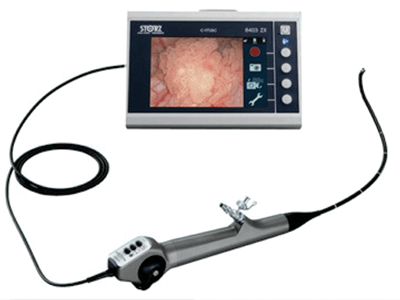Figure A.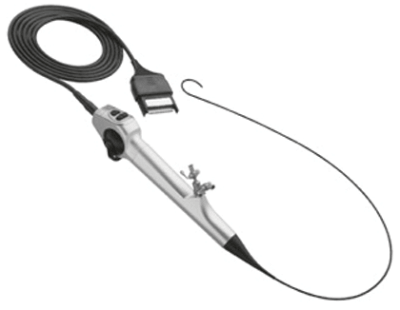Figure B.

1. What is shown in figures A and B?
2. What is the diameter of each scope?
3. What is the working channel size of each?
4. What are the advantages of a digital versus optic flexible ureteroscope?

## Case 2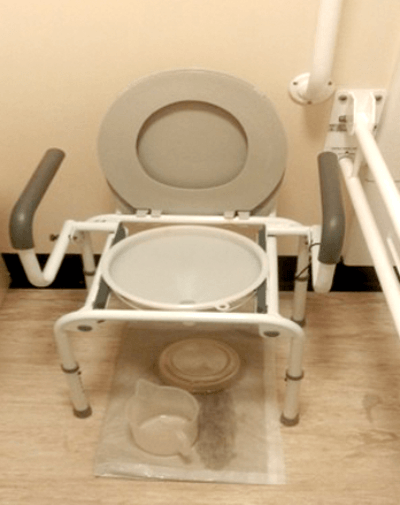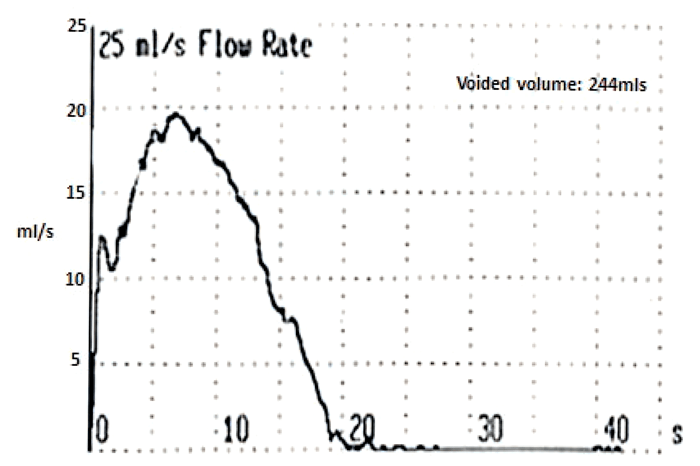1. What is this machine?
2. What are the different methods of its operation?
3. Calculate the following uroflow parameters: voided volume (Vcomp), voiding time (T100), maximum flow (Qmax).
4. What is the probability of obstruction with a Q-max of <10ml/s, 10-15ml/s and >15ml/s?

## Case 3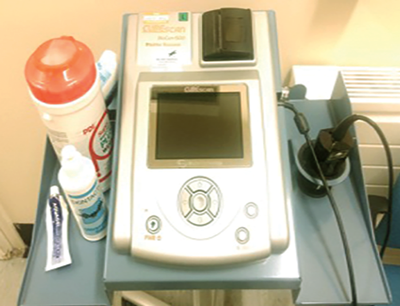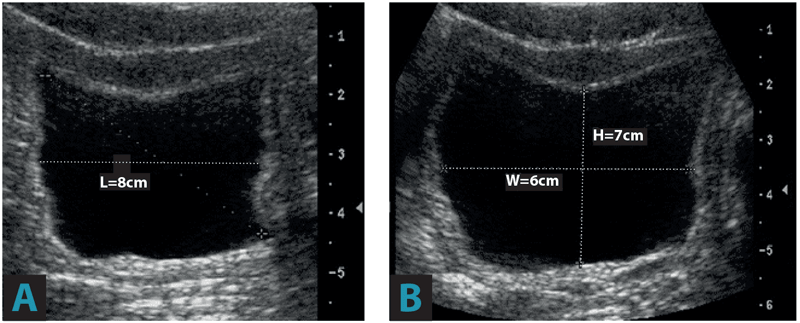1. What is this machine?
2. How does it calculate volume?
3. Calculate the urine volume from the ultrasound images A and B.